## Ambiguous Grammar & Parse Tree-

We have discussed-

• Ambiguous Grammar generates at least one string that has more than one parse tree.
• Parse Tree is the geometrical representation of a derivation.

## Important Points-

### Point-01:

• There always exists a unique parse tree corresponding to each leftmost derivation and rightmost derivation.

 If n parse trees exist for any string w, then there will be-n corresponding leftmost derivations.n corresponding rightmost derivations.

### Point-02:

For ambiguous grammars,

• More than one leftmost derivation and more than one rightmost derivation exist for at least one string.
• Leftmost derivation and rightmost derivation represents different parse trees.

### Point-03:

For unambiguous grammars,

• A unique leftmost derivation and a unique rightmost derivation exist for all the strings.
• Leftmost derivation and rightmost derivation represents the same parse tree.

### Point-04:

• There may exist derivations for a string which are neither leftmost nor rightmost.

### Example

Consider the following grammar-

S → ABC

A → a

B → b

C → c

Consider a string w = abc.

Total 6 derivations exist for string w.

The following 4 derivations are neither leftmost nor rightmost-

#### Derivation-01:

S → ABC

→ aBC    (Using A → a)

→ aBc     (Using C → c)

→ abc     (Using B → b)

#### Derivation-02:

S → ABC

→ AbC    (Using B → b)

→ abC     (Using A → a)

→ abc     (Using C → c)

#### Derivation-03:

S → ABC

→ AbC    (Using B → b)

→ Abc     (Using C → c)

→ abc     (Using A → a)

The other 2 derivations are leftmost derivation and rightmost derivation.

### Point-05:

• Leftmost derivation and rightmost derivation of a string may be exactly same.
• In fact, there may exist a grammar in which leftmost derivation and rightmost derivation is exactly same for all the strings.

### Example

Consider the following grammar-

S → aS / ∈

The language generated by this grammar is-

L = { an , n>=0 } or a*

All the strings generated from this grammar have their leftmost derivation and rightmost derivation exactly same.

Let us consider a string w = aaa.

#### Leftmost Derivation-

S → aS

→ aaS       (Using S → aS)

→ aaaS     (Using S → aS)

→ aaa∈

→ aaa

#### Rightmost Derivation-

S → aS

→ aaS       (Using S → aS)

→ aaaS     (Using S → aS)

→ aaa∈

→ aaa

Clearly,

Leftmost derivation = Rightmost derivation

Similar is the case for all other strings.

### Point-06:

• For a given parse tree, we may have its leftmost derivation exactly same as rightmost derivation.

### Point-07:

• If for all the strings of a grammar, leftmost derivation is exactly same as rightmost derivation, then that grammar may be ambiguous or unambiguous.

### Example

Consider the following grammar-

S → aS / ∈

• This is an example of an unambiguous grammar.
• Here, each string have its leftmost derivation and rightmost derivation exactly same.

Now, consider the following grammar-

S → aS / a / ∈

• This is an example of ambiguous grammar.
• Here also, each string have its leftmost derivation and rightmost derivation exactly same.

Consider a string w = a.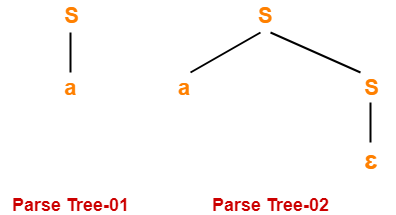Since two different parse trees exist, so grammar is ambiguous.

Leftmost derivation and rightmost derivation for parse tree-01 are-

S → a

Leftmost derivation and rightmost derivation for parse tree-02 are-

S → aS

S → a∈

S → a

Next Article- Language of Grammar

Get more notes and other study material of Theory of Automata and Computation.

Watch video lectures by visiting our YouTube channel LearnVidFun.

## Grammar Ambiguity-

Before you go through this article, make sure that you have gone through the previous article on Grammar Ambiguity.

We have discussed-

• There exists no general algorithm to remove the ambiguity from grammar.
• To check grammar ambiguity, we try finding a string that has more than one parse tree.
• If any such string exists, then the grammar is ambiguous otherwise not.

In this article, we will discuss how to convert ambiguous grammar into unambiguous grammar.

## Converting Ambiguous Grammar Into Unambiguous Grammar-

• Causes such as left recursion, common prefixes etc makes the grammar ambiguous.
• The removal of these causes may convert the grammar into unambiguous grammar.
•  However, it is not always compulsory.

### NOTE

It is not always possible to convert an ambiguous grammar into an unambiguous grammar.

## Methods To Remove Ambiguity-

The ambiguity from the grammar may be removed using the following methods-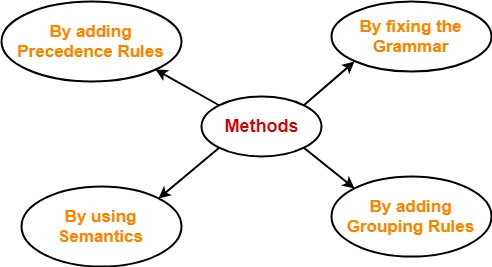• By fixing the grammar
• By using semantics and choosing the parse that makes the most sense
• By adding the precedence rules or other context sensitive parsing rules

## Removing Ambiguity By Precedence & Associativity Rules-

An ambiguous grammar may be converted into an unambiguous grammar by implementing-

• Precedence Constraints
• Associativity Constraints

These constraints are implemented using the following rules-

### Rule-01:

The precedence constraint is implemented using the following rules-

• The level at which the production is present defines the priority of the operator contained in it.
• The higher the level of the production, the lower the priority of operator.
• The lower the level of the production, the higher the priority of operator.

### Rule-02:

The associativity constraint is implemented using the following rules-

• If the operator is left associative, induce left recursion in its production.
• If the operator is right associative, induce right recursion in its production.

## Problem-01:

Convert the following ambiguous grammar into unambiguous grammar-

R → R + R / R . R / R* / a / b

where * is kleen closure and . is concatenation.

## Solution-

To convert the given grammar into its corresponding unambiguous grammar, we implement the precedence and associativity constraints.

We have-

• Given grammar consists of the following operators-

+ , . , *

• Given grammar consists of the following operands-

a , b

The priority order is-

(a , b) > * > . > +

where-

• . operator is left associative
• + operator is left associative

Using the precedence and associativity rules, we write the corresponding unambiguous grammar as-

E → E + T / T

T → T . F / F

F → F* / G

G → a / b

Unambiguous Grammar

OR

E → E + T / T

T → T . F / F

F → F* / a / b

Unambiguous Grammar

## Problem-02:

Convert the following ambiguous grammar into unambiguous grammar-

bexp → bexp or bexp / bexp and bexp / not bexp / T / F

where bexp represents Boolean expression, T represents True and F represents False.

## Solution-

To convert the given grammar into its corresponding unambiguous grammar, we implement the precedence and associativity constraints.

We have-

• Given grammar consists of the following operators-

or , and , not

• Given grammar consists of the following operands-

T , F

The priority order is-

(T , F) > not > and > or

where-

• and operator is left associative
• or operator is left associative

Using the precedence and associativity rules, we write the corresponding unambiguous grammar as-

bexp → bexp or M / M

M → M and N / N

N → not N / G

G → T / F

Unambiguous Grammar

OR

bexp → bexp or M / M

M → M and N / N

N → not N / T / F

Unambiguous Grammar

To gain better understanding about Conversion into Unambiguous Grammar-

Watch this Video Lecture

Next Article- Evaluating Expressions Based On Given Grammar

Get more notes and other study material of Theory of Automata and Computation.

Watch video lectures by visiting our YouTube channel LearnVidFun.

## Evaluating Expressions Based On Given Grammar-

There are two methods for evaluating the expressions based on given grammar-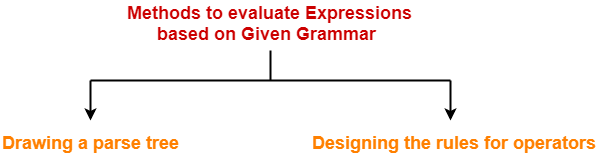1. Drawing a parse tree
2. Designing the rules for operators

### Method-01: Drawing Parse Tree-

In this method, following steps are followed-

• We draw a Parse Tree for the given expression.
• We evaluate the parse tree to get the required value of the expression.

### Drawback-

• Drawing a parse tree for large expressions is cumbersome and time taking.
• So, this method becomes difficult to follow when the expression is large.

### Method-02: Designing Rules For Operators-

In this method, following steps are followed-

• We decide the priority and associativity of operators in the grammar.
• We parenthesize the given expression.
• We evaluate the expression to get the required value.

• This method is easy to follow whether the expression is small or large.
• So, this method is preferred.

### Rules For Deciding Priority Of Operators-

For the given unambiguous grammar,

• The priority of operators is decided by checking the level at which the production is present.
• The higher the level of production, the lower the priority of operator contained in it.
• The lower the level of production, the higher the priority of operator contained in it.

### Example-

Consider the grammar-

E → E x F / F + E / F

F → F – T / T

T → id

Here,

• id has the highest priority.

(since T → id is present at the bottom most level)

• x and + operators have the least priority.

(since E → E x F / F + E are present at the top most level)

• x and + operators have the same priority.

(since E → E x F / F + E are present at the same level)

• – operator has higher priority than x and + but lesser priority than id.

(since F → F – T is present at the middle level)

So, the priority order is-

id > ( x , + )

### Rules For Deciding Associativity Of Operators-

For the given unambiguous grammar,

• The associativity of operators is decided by checking the Type Of Recursion in the production.
• If the production has left recursion, then the operator is left associative.
• If the production has right recursion, then the operator is right associative.
• If the production has both left and right recursion, then the operator is neither left associative nor right associative.

### Example-

Consider the grammar-

E → E x F / F + E / F

F → F – F / T

T → id

Here,

• x operator is left associative.

(since E → E x F has left recursion in it)

• + operator is right associative.

(since E → F + E has right recursion in it)

• F – F is neither left associative nor right associative.

(since F → F – F has both left and right recursion in it)

## Problem-01:

Consider the given grammar-

E → E + T / T

T → F x T / F

F → id

Evaluate the following expression in accordance with the given grammar-

2 + 3 x 5 x 6 + 2

## Solution-

### Method-01:

• Let us draw a parse tree for the given expression.
• Evaluating the parse tree will return the required value of the expression.

The parse tree for the given expression is-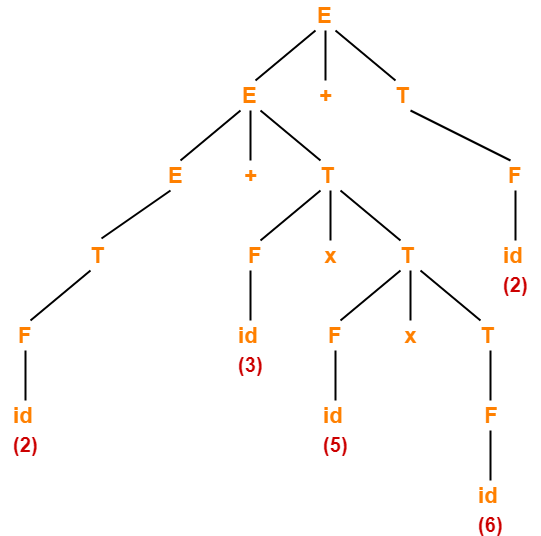On evaluating this parse tree, we get the value = 94.

### Method-02:

The priority order and associativity of operators on the basis of given grammar is-

idx > +

where-

• x operator is right associative.
• + operator is left associative.

Now, we parenthesize the given expression based on the precedence and associativity of operators as-

( 2 + ( 3 x ( 5 x 6 ) ) ) + 2

Now, we evaluate the parenthesized expression as-

= ( 2 + ( 3 x ( 5 x 6 ) ) ) + 2

= ( 2 + ( 3 x 30 ) ) + 2

= ( 2 + 90 ) + 2

= 92 + 2

= 94

## Problem-02:

Consider the given grammar-

E → E + T / E – T / T

T → T x F / T ÷ F / F

F → G ↑ F / G

G → id

Evaluate the following expression in accordance with the given grammar-

2 x 1 + 4 ↑ 2 ↑ 1 x 1 + 3

## Solution-

### Method-01:

• Let us draw a parse tree for the given expression.
• Evaluating the parse tree will return the required value of the expression.

The parse tree for the given expression is-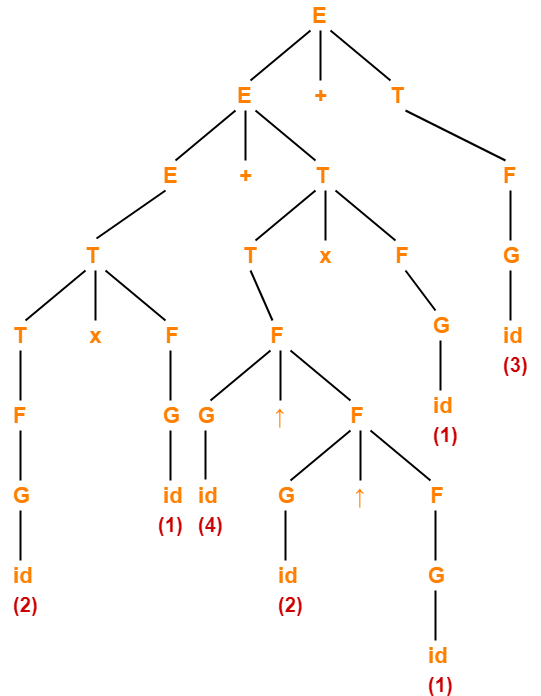On evaluating this parse tree, we get the value = 21.

### Method-02:

The priority order and associativity of operators on the basis of given grammar is-

id > ( x÷ ) > ( + , )

where-

• + , – , x , ÷ operators are left associative.
• ↑ operator is right associative

Now, we parenthesize the given expression based on the precedence and associativity of operators as-

( ( 2 x 1 ) + ( ( 4 ↑ ( 2 ↑ 1 ) ) x 1 ) ) + 3

Now, we evaluate the parenthesized expression as-

= ( ( 2 x 1 ) + ( ( 4 ↑ ( 2 ↑ 1 ) ) x 1 ) ) + 3

= ( ( 2 x 1 ) + ( ( 4 ↑ 2 ) x 1 ) ) + 3

= ( ( 2 x 1 ) + ( 16 x 1 ) ) + 3

= ( 2 + ( 16 x 1 ) ) + 3

( 2 + 16 ) + 3

= 18 + 3

= 21

## Problem-03:

Consider the given grammar-

E → E + T / E – T / T

T → T x F / T ÷ F / F

F → F ↑ G / G

G → id

Evaluate the following expression in accordance with the given grammar-

2 ↑ 1 ↑ 4 + 3 x 5 x 6 ↑ 1 + 2 ↑ 3

## Solution-

The priority order and associativity of operators on the basis of given grammar is-

id > ( x÷ ) > ( + , )

where + , – , x , ÷, ↑ operators are left associative.

Now, we parenthesize the given expression based on the precedence and associativity of operators as-

( ( ( 2 ↑ 1 ) ↑ 4 ) + ( ( 3 x 5 ) x ( 6 ↑ 1 ) ) ) + ( 2 ↑ 3 )

Now, we evaluate the parenthesized expression as-

= ( ( ( 2 ↑ 1 ) ↑ 4 ) + ( ( 3 x 5 ) x ( 6 ↑ 1 ) ) ) + ( 2 ↑ 3 )

= ( ( 2 ↑ 4 ) + ( ( 3 x 5 ) x ( 6 ↑ 1 ) ) ) + ( 2 ↑ 3 )

= ( 16 + ( ( 3 x 5 ) x ( 6 ↑ 1 ) ) ) + ( 2 ↑ 3 )

= ( 16 + ( ( 3 x 5 ) x 6 ) ) + ( 2 ↑ 3 )

= ( 16 + ( ( 3 x 5 ) x 6 ) ) + 8

= ( 16 + ( 15 x 6 ) ) + 8

( 16 + 90 ) + 8

= 106 + 8

= 114

## Problem-04:

Consider the given grammar-

E → E ↑ T / T

T → T + F / F

F → G – F / G

G → id

Evaluate the following expression in accordance with the given grammar-

2 ↑ 1 ↑ 3 + 5 – 6 – 8 – 5 + 10 + 11 ↑ 2

## Solution-

The priority order and associativity of operators on the basis of given grammar is-

id >  > + >

where-

• + , ↑ operators are left associative.
• – is right associative.

Now, we parenthesize the given expression based on the precedence and associativity of operators as-

( ( 2 ↑ 1 ) ↑ ( ( ( 3 + ( 5 – ( 6 – ( 8 – 5 ) ) ) ) + 10 ) + 11 ) ) ↑ 2

Now, we evaluate the parenthesized expression as-

= ( ( 2 ↑ 1 ) ↑ ( ( ( 3 + ( 5 – ( 6 – ( 8 – 5 ) ) ) ) + 10 ) + 11 ) ) ↑ 2

= ( ( 2 ↑ 1 ) ↑ ( ( ( 3 + ( 5 – ( 6 – 3 ) ) ) + 10 ) + 11 ) ) ↑ 2

= ( ( 2 ↑ 1 ) ↑ ( ( ( 3 + ( 5 – 3 ) ) + 10 ) + 11 ) ) ↑ 2

= ( ( 2 ↑ 1 ) ↑ ( ( ( 3 + 2 ) + 10 ) + 11 ) ) ↑ 2

= ( ( 2 ↑ 1 ) ↑ ( ( 5 + 10 ) + 11 ) ) ↑ 2

= ( ( 2 ↑ 1 ) ↑ ( 15 + 11 ) ) ↑ 2

= ( ( 2 ↑ 1 ) ↑ 26 ) ↑ 2

= ( 2 ↑ 26 ) ↑ 2

= (226) ↑ 2

= (226)2

## Problem-05:

Consider the given priority order of operators-

id > ( x÷ ) > ( + , )

Given all the operators are left associative, construct the corresponding grammar.

## Solution-

We apply the rules learnt above in reverse direction to obtain the required grammar.

The corresponding grammar is-

E → E + T / E – T / T

T → T x F / T ÷ F / F

F → id

## Problem-06:

Consider the given priority order of operators-

id > ( x÷ ) > ( + , )

Given all the operators are left associative, construct the corresponding grammar.

## Solution-

The corresponding grammar is-

E → E + T / E – T / T

T → T x F / T ÷ F / F

F → G ↑ F / G

G → id

Next Article- Important Points For Exams

Get more notes and other study material of Theory of Automata and Computation.

Watch video lectures by visiting our YouTube channel LearnVidFun.

## Normal Forms-

 Normalization is performed in order to standardize the grammar.

• By reducing the grammar, the grammar gets minimized but does not gets standardized.
• This is because the RHS of productions have no specific format.
• In order to standardize the grammar, normalization is performed using normal forms.

## Types of Normal Forms-

The most frequently used normal forms are-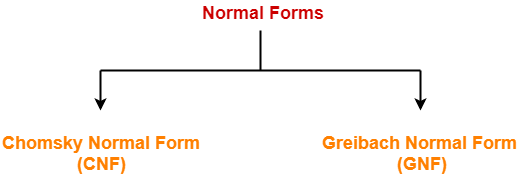1. Chomsky Normal Form (CNF)
2. Greibach Normal Form (GNF)

## Chomsky Normal Form-

 A context free grammar is said to be in chomsky normal form (CNF) if all its productions are of the form-A → BC or A → awhere A, B, C are non-terminals and a is a terminal.

From here, we infer-

• To be in CNF, all the productions must derive either two non-terminals or a single terminal.
• CNF restricts the number of symbols on the right side of a production to be two.
• The two symbols must be non-terminals or a single terminal.

### Example-

S → AB

A → a

B → b

This context free grammar is in chomsky normal form.

## Steps-

The following steps are followed to standardize the grammar using CNF-

### Rule-01:

Reduce the grammar completely by-

• Eliminating ∈ productions
• Eliminating unit productions
• Eliminating useless productions

Also Watch- How To Reduce Grammar?

### Rule-02:

Replace each production of the form A → B1B2B3….Bn where n > 2 with A → B1C where C → B2B3….Bn.

Repeat this step for all the productions having more than two variables on RHS.

### Rule-03:

Replace each production of the form A → aB with A → XB and X → a.

Repeat this step for all the productions having the form A → aB.

## Problem-01:

Convert the given grammar to CNF-

A → aB / bAB

B → b

D → d

## Solution-

### Step-01:

The given grammar is already completely reduced.

### Step-02:

The productions already in chomsky normal form are-

B → b        ………..(1)

D → d        ………..(2)

These productions will remain as they are.

The productions not in chomsky normal form are-

A → aB / bAB         ………..(4)

We will convert these productions in chomsky normal form.

### Step-03:

Replace the terminal symbols a and b by new variables Ca and Cb.

This is done by introducing the following two new productions in the grammar-

Ca → a       ………..(5)

Cb → b       ………..(6)

Now, the productions (3) and (4) modifies to-

A → CaB / CbAB         ………..(8)

### Step-04:

This is done by introducing the following two new productions in the grammar-

CAB → AB       ………..(10)

Now, the productions (7) and (8) modifies to-

A → CaB / CbCAB         ………..(12)

### Step-05:

From (1), (2), (5), (6), (9), (10), (11) and (12), the resultant grammar is-

A → CaB / CbCAB

B → b

D → d

Ca → a

Cb → b

CAB → AB

This grammar is in chomsky normal form.

## Problem-02:

Convert the given grammar to CNF-

S → 1A / 0B

A → 1AA / 0S / 0

B → 0BB / 1S / 1

## Solution-

### Step-01:

The given grammar is already completely reduced.

### Step-02:

The productions already in chomsky normal form are-

A → 0        ………..(1)

B → 1        ………..(2)

These productions will remain as they are.

The productions not in chomsky normal form are-

S → 1A / 0B           ………..(3)

A → 1AA / 0S         ………..(4)

B → 0BB / 1S         ………..(5)

We will convert these productions in chomsky normal form.

### Step-03:

Replace the terminal symbols 0 and 1 by new variables C and D.

This is done by introducing the following two new productions in the grammar-

C → 0       ………..(6)

D → 1       ………..(7)

Now, the productions (3), (4) and (5) modifies to-

S → DA / CB           ………..(8)

A → DAA / CS         ………..(9)

B → CBB / DS         ………..(10)

### Step-04:

Out of (8), (9) and (10), the productions already in Chomsky Normal Form are-

S → DA / CB           ………..(11)

A → CS                   ………..(12)

B → DS                   ………..(13)

These productions will remain as they are.

The productions not in chomsky normal form are-

A → DAA         ………..(14)

B → CBB         ………..(15)

We will convert these productions in Chomsky Normal Form.

### Step-05:

Replace AA and BB by new variables E and F respectively.

This is done by introducing the following two new productions in the grammar-

E → AA       ………..(16)

F → BB       ………..(17)

Now, the productions (14) and (15) modifies to-

A → DE         ………..(18)

B → CF         ………..(19)

### Step-06:

From (1), (2), (6), (7), (11), (12), (13), (16), (17), (18) and (19), the resultant grammar is-

S → DA / CB

A → CS / DE / 0

B → DS / CF / 1

C → 0

D → 1

E → AA

F → BB

This grammar is in chomsky normal form.

To gain better understanding about Chomsky Normal Form,

Watch this Video Lecture

Next Article- Algorithm To Decide Whether CFL Is Empty

Get more notes and other study material of Theory of Automata and Computation.

Watch video lectures by visiting our YouTube channel LearnVidFun.

## Minimization of DFA-

 The process of reducing a given DFA to its minimal form is called as minimization of DFA.

• It contains the minimum number of states.
• The DFA in its minimal form is called as a Minimal DFA.

## How To Minimize DFA?

The two popular methods for minimizing a DFA are-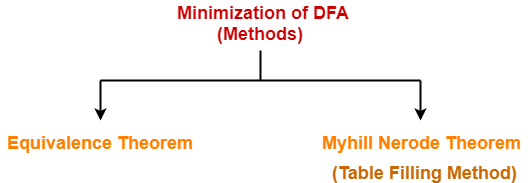In this article, we will discuss Minimization of DFA Using Equivalence Theorem.

## Minimization of DFA Using Equivalence Theorem-

### Step-01:

• Eliminate all the dead states and inaccessible states from the given DFA (if any).

All those non-final states which transit to itself for all input symbols in ∑ are called as dead states.

### Inaccessible State

All those states which can never be reached from the initial state are called as inaccessible states.

### Step-02:

• Draw a state transition table for the given DFA.
• Transition table shows the transition of all states on all input symbols in Σ.

### Step-03:

Now, start applying equivalence theorem.

• Take a counter variable k and initialize it with value 0.
• Divide Q (set of states) into two sets such that one set contains all the non-final states and other set contains all the final states.
• This partition is called P0.

### Step-04:

• Increment k by 1.
• Find Pk by partitioning the different sets of Pk-1 .
• In each set of Pk-1 , consider all the possible pair of states within each set and if the two states are distinguishable, partition the set into different sets in Pk.

 Two states q1 and q2 are distinguishable in partition Pk for any input symbol ‘a’,if δ (q1, a) and δ (q2, a) are in different sets in partition Pk-1.

### Step-05:

• Repeat step-04 until no change in partition occurs.
• In other words, when you find Pk = Pk-1, stop.

### Step-06:

• All those states which belong to the same set are equivalent.
• The equivalent states are merged to form a single state in the minimal DFA.

 Number of states in Minimal DFA= Number of sets in Pk

## Problem-01:

Minimize the given DFA-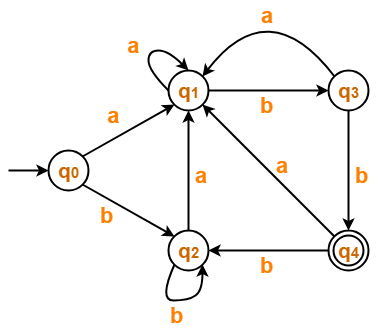## Solution-

### Step-01:

The given DFA contains no dead states and inaccessible states.

### Step-02:

Draw a state transition table-

 a b →q0 q1 q2 q1 q1 q3 q2 q1 q2 q3 q1 *q4 *q4 q1 q2

### Step-03:

Now using Equivalence Theorem, we have-

P0 = { q0 , q1 , q2 , q3 } { q4 }

P1 = { q0 , q1 , q2 } { q3 } { q4 }

P2 = { q0 , q2 } { q1 } { q3 } { q4 }

P3 = { q0 , q2 } { q1 } { q3 } { q4 }

Since P3 = P2, so we stop.

From P3, we infer that states q0 and q2 are equivalent and can be merged together.

So, Our minimal DFA is-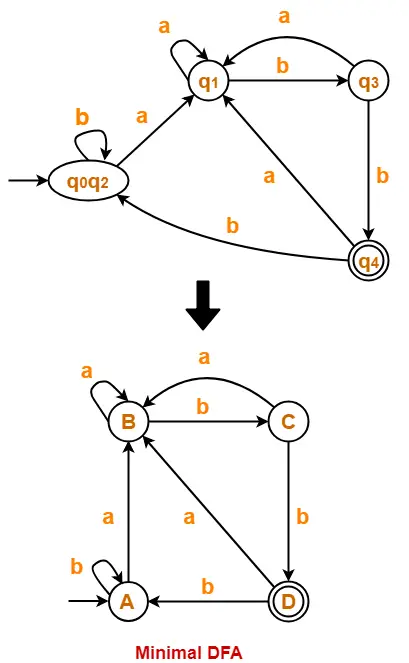## Problem-02:

Minimize the given DFA-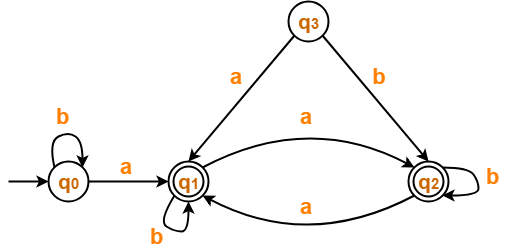## Solution-

### Step-01:

• State q3 is inaccessible from the initial state.
• So, we eliminate it and its associated edges from the DFA.

The resulting DFA is-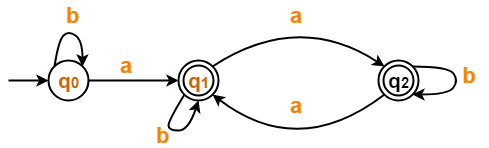### Step-02:

Draw a state transition table-

 a b →q0 *q1 q0 *q1 *q2 *q1 *q2 *q1 *q2

### Step-03:

Now using Equivalence Theorem, we have-

P0 = { q0 } { q1 , q2 }

P1 = { q0 } { q1 , q2 }

Since P1 = P0, so we stop.

From P1, we infer that states q1 and q2 are equivalent and can be merged together.

So, Our minimal DFA is-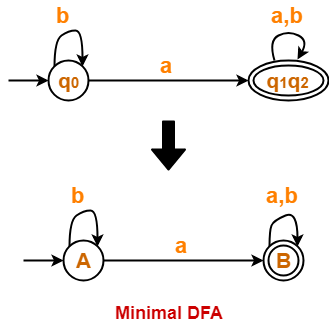## Problem-03:

Minimize the given DFA-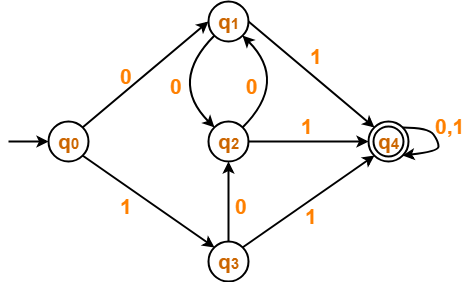## Solution-

### Step-01:

The given DFA contains no dead states and inaccessible states.

### Step-02:

Draw a state transition table-

 0 1 →q0 q1 q3 q1 q2 *q4 q2 q1 *q4 q3 q2 *q4 *q4 *q4 *q4

### Step-03:

Now using Equivalence Theorem, we have-

P0 = { q0 , q1 , q2 , q3 } { q4 }

P1 = { q0 } { q1 , q2 , q3 } { q4 }

P2 = { q0 } { q1 , q2 , q3 } { q4 }

Since P2 = P1, so we stop.

From P2, we infer that states q1 , q2 and q3 are equivalent and can be merged together.

So, Our minimal DFA is-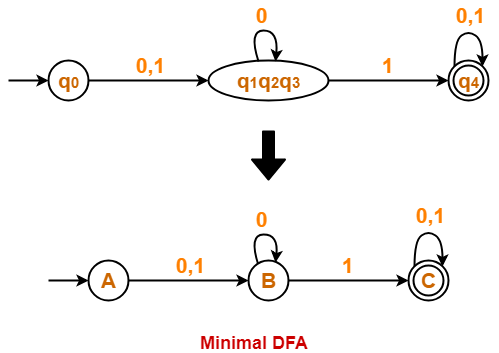## Problem-04:

Minimize the given DFA-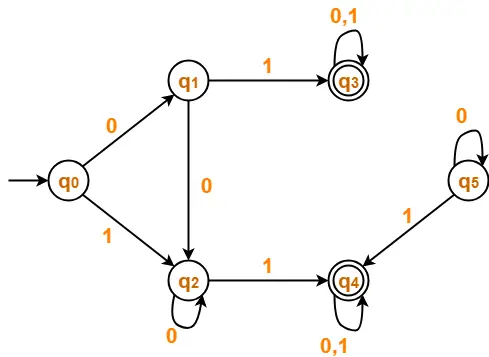## Solution-

### Step-01:

• State q5 is inaccessible from the initial state.
• So, we eliminate it and its associated edges from the DFA.

The resulting DFA is-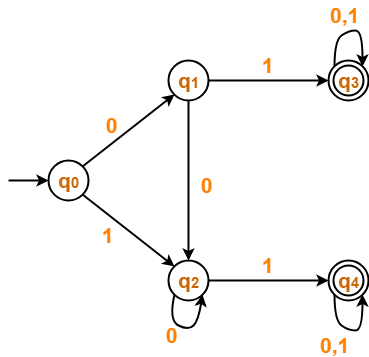### Step-02:

Draw a state transition table-

 0 1 →q0 q1 q2 q1 q2 *q3 q2 q2 *q4 *q3 *q3 *q3 *q4 *q4 *q4

### Step-03:

Now using Equivalence Theorem, we have-

P0 = { q0 , q1 , q2 } { q3 , q4 }

P1 = { q0 } { q1 , q2 } { q3 , q4 }

P2 = { q0 } { q1 , q2 } { q3 , q4 }

Since P2 = P1, so we stop.

From P2, we infer-

• States q1 and q2 are equivalent and can be merged together.
• States q3 and q4 are equivalent and can be merged together.

So, Our minimal DFA is-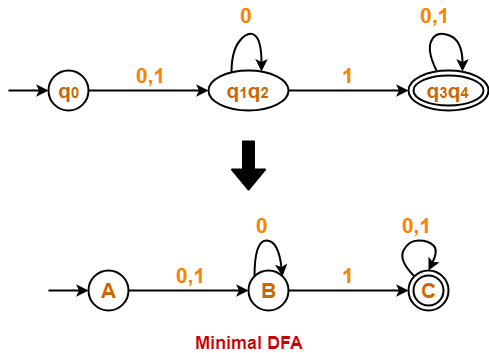To gain better understanding about Minimization of DFA,

Watch this Video Lecture

Next Article- Converting DFA to Regular Expression

Get more notes and other study material of Theory of Automata and Computation.

Watch video lectures by visiting our YouTube channel LearnVidFun.